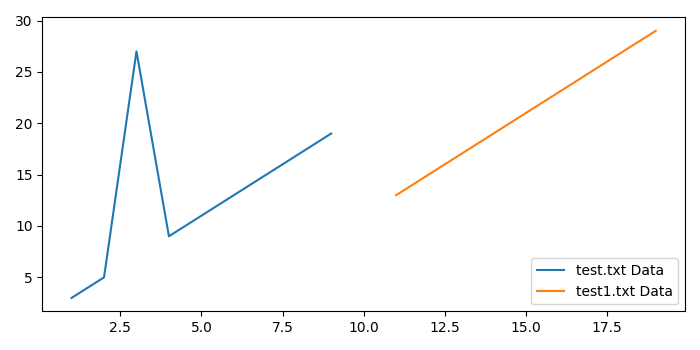# How to plot data from multiple two-column text files with legends in Matplotlib?

To plot data from multiple two-column text files with legends in matplotlib, we can take the following steps −

• Import genfromtxt from pylab. It has several options to read data from a text file and plot the data.

• Read two text files, test.txt and test1.txt (having two columns of data), using genfromtxt and store the data in two variables, firstfiledata and secondfiledata.

• Plot the data using plot() method. label will be displayed as the legend.

• To display the figure, use show() method.

## Example

from matplotlib import pyplot as plt;
from pylab import genfromtxt;

plt.rcParams["figure.figsize"] = [7.00, 3.50]
plt.rcParams["figure.autolayout"] = True

firstfiledata = genfromtxt("test.txt");
secondfiledata = genfromtxt("test1.txt");
plt.plot(firstfiledata[:, 0], firstfiledata[:, 1], label="test.txt Data");
plt.plot(secondfiledata[:, 0], secondfiledata[:, 1], label="test1.txt Data");
plt.legend();
plt.show();

## Output

Suppse the two text files have the following data −

test.txt
========
1    3
2    5
3    27
4    9
5    11
6    13
7    15
8    17
9    19

test1.txt
=========
11    13
12    15
13    17
14    19
15    21
16    23
17    25
18    27
19    29

When we execute the code, it will produce the following output −